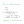0%
0%

# µδ code

A binary maths hack, for use in DSP code and lossless compression

Similar projects worth following
163 views
For your DSP/DCT/FFT needs, µδ codes are the next enhancement, beyond the lifting scheme and dyadic multiplies.
As far as I know, it has not yet been documented openly or used because most of the research is focused on lossy compression (think: MPEG).
µδ codes make DSP algos more bit-efficient and will be used in my lossless video and audio CODECs, along with the 3R compaction algorithm ( https://hackaday.io/project/7385-recursive-range-reduction-3r-hwsw-codec )
More details will appear here soon :-)

A µδ CODEC adds only one XOR gate to the critical datapath of a circuit that computes the simultaneous sum and difference of two integer numbers. The transform is perfectly bit-reversible and the reverse transform is almost identical to the classic version.

The two results (µ and δ) are as large as the original numbers, whereas a classical sum&difference expands the data by 2 bits (yet the result can only use 1/4 of the coding space).

The results are distorted : the MSB of µ gets disturbed by the sign of δ, which is simply truncated. The bet is that this distorsion is not critical for certain types of lossless compression CODECs, while reducing the size and consumption of hardware circuits.

Yann Guidon / YGDES05/24/2017 at 18:12 0 comments

Look at these new logs:

The have some similarities with µδ but they differ in critical ways:

• µδ works with median and difference while the new transform creates a sum (σ) and one of the operands (let's call it α). The new transform shall then called σα.
• µδ works inside a ring (a closed set with wrap-around) but σα "escapes" this (the output is not constrained inside the original boundaries), which allows actual compression for some combinations of values.
• actually σα has an initial value range that is a ring, however each operand can have its own original ring, they both are combined and the boundaries remain as auxiliary data in the background.
• σα is used with VLC (variable length codes) while µδ uses fixed-length words.

σα already has a fantastic application in the color decomposition of RGB pictures and will make 3R a truly entropy coder... Follow the developments there !

• ### X+X = 2X

Yann Guidon / YGDES07/09/2016 at 16:01 0 comments

Before we start, let's just remember and examine one of the fundamental rules of arithmetics. If you take 2 non-negative numbers and add them, the sum is equal or greater than any of the addends. This is the rule that makes the #Recursive Range Reduction (3R) HW&SW CODEC work.

In a more general way, if you have two numbers of N bits, you need N+1 bits to uniquely represent the sum. Since X+X=2X, 2^N + 2^N = 2^(N+1). This +1 is the carry bit and is required to restore the original addends if you ever want to subtract it back from the other.

Same goes for the subtraction : the difference requires a "borrow" bit. You can't avoid this, even though there are some cases where you can work modulo 2^N.

We are used to dealing with the carry and borrow bits but things can quickly get out of hand! Imagine you want to compute a 1024-tap integer FFT: each result will be the sum of 2^10 numbers, adding 10 bits to the original sample size. If you're dealing with CD quality, 16+10=26 bits so it fits in the 32-bits registers of common CPUs or DSPs.

Now if you want to use 24-bits samples, you're screwed. 34 bits don't easily fit in 32-bits registers. Will you resort to slower 32-bits floating points ? 40-bits integers ? 64-bits integers ?

Take the classical 8×8 DCT square now. The original 8-bits samples of a picture get fatter during each of the 3+3=6 passes, resulting in 14 bits for the results. The integer units have almost doubled the precision of the source data and this considerably increases gate count, latency, power consumption...

Now you start to see where I'm getting to : the classical filters and time-frequency transforms have a fundamental problem of size.

• ### First publication

Yann Guidon / YGDES07/08/2016 at 08:11 8 comments

OpenSilicium#19 is out !

I'll publish the code soon.

• 1
Step 1

# Step 1: encoding

• Take A and B, two signed numbers made of N bits
• Compute the sum µ = A + B (with N+1 resolution)
• Compute the difference δ = A - B (with N+1 bits of resolution)
• Adjust the sign bit : µ = µ xor MSB(δ)
• Remove the LSB of µ : µ = µ shr 1 (µ now uses N bits)
• Remove the MSB of δ : δ = δ mod 2^N (δ now uses N bits)
• 2
Step 2

# Step 2: decoding

• Take the coded values µ and δ, with N bits each
• Restore the LSB of µ by copying it from δ: µ = (µ shl 1) + (δ mod 2)
• Restore A but drop the MSB: A = (µ + δ) mod 2^N
• Restore B but drop the MSB: B = (µ - δ) mod 2^N

Share

## Discussions

Yann Guidon / YGDES wrote 06/30/2016 at 16:31 point

The founding article will be published in french kiosks in a few days now :-) I can't wait !

Are you sure? yes | no

## Similar Projects

Project Owner Contributor

### Recursive Range Reduction (3R) HW&SW CODECYann Guidon / YGDES

Project Owner Contributor

### 3RGB image lossless compression formatYann Guidon / YGDES

# Does this project spark your interest?

Become a member to follow this project and never miss any updates